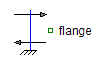Multiphase Quasi-RMS Potential Sensor - MapleSim Help

Home : Support : Online Help : MapleSim : MapleSim Component Library : Electrical : Multiphase Systems : Sensors : Multiphase Quasi-RMS Potential Sensor

Potential Quasi-RMS Sensor

Multiphase quasi-rms potential sensorDescription The Potential Quasi-RMS Sensor component measures the continuous quasi-RMS value of a multiphase potential. If the potential waveform deviates from a sine curve, the output of the sensor will not be exactly the average RMS value.Equations ${V}_{\mathrm{rms}}=\sqrt{\frac{1}{m}\sum _{k=1}^{m}{\mathrm{\phi }}_{k}^{2}}$ $i={i}_{p}=0$ $\mathrm{\phi }={v}_{p}$Connections

 Name Description Modelica ID ${\mathrm{plug}}_{p}$ Positive $m$-phase plug plug_p $V$ Real output; quasi-RMS of potential in $V$ VConstants

 Name Value Units Description Modelica ID $m$ $3$ Number of phases mModelica Standard Library The component described in this topic is from the Modelica Standard Library. To view the original documentation, which includes author and copyright information, click here.